Algebraic Translations MathBitsNotebook.com Terms of Use   Contact Person: Donna RobertsAlgebraic Expressions:

Unlike equations, algebraic expressions do not contain an equal sign.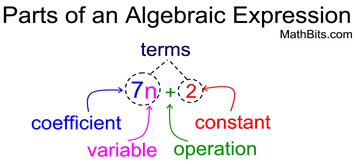The word "terms" refers to values that are added or subtracted. 7n + 2 has two terms. The word "factors" refers to values that are multiplied. The term 7n has two factors: 7 and n.

The skill of translating between verbal statements and algebraic expressions requires an understanding of the words that are commonly used to represent mathematical operations.

A sampling of words used to represent mathematical operations:
 ADD SUBTRACT MULTIPLY DIVIDE add sum increased by more than exceeds total plus in all gain deposit subtract difference decreased by fewer less than * diminished by minus take away withdraw reduced by multiply product of times double triple twice divide quotient per divided equally split into fraction ratio of
* Remember that "less than" reverses the order of what you read. [ 6 less than x, becomes x - 6 ]Always look for the placement of commas in the verbal statements. They will help you decide how to properly group terms in your algebraic expression. For example:  the verbal statement "5 times, a number increased by 6" translates to 5(n + 6) and is not 5n + 6.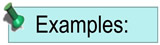(Remember that variables can be represented by any letter or symbol, unless otherwise directed.)
 Verbal Statement Algebraic Expression 1. Five increased by four times a number 5 + 4n 2. Eight less than twice a number 2n - 8 3. Three times a number, increased by 9 3n + 9 4. The product of 4, and a number decreased by 7 4(n - 7) 5. The number of feet in x yards. 3x 6. Express the width of a rectangle which is seven less than its length, l. l - 7 7. A number repeated as a factor 3 times. n • n • n = n3

Terms used to refer to specific expressions:

 TERM DESCRIPTION EXAMPLES monomial The product of non-negative integer powers of variables. It has ONE term. (mono implies one) 12, -3x, 2x2, -7ab, 4t3s4 binomial The sum of two monomials. It has TWO unlike terms. (bi implies two) 7x + 4,  x2 +1,  3a - 2b trinomial The sum of three monomials. It has THREE unllike terms. (tri implies three) x2 + 4x + 4,  4p2 - 3q3 - 1 polynomial A generic term for the sum of one or more monomials. (poly implies many) 4x,  s + 2,  a2 + b2 + cAlgebraic Equations:

Unlike expressions, algebraic equations contain an equal sign.
EQUA
tion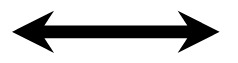EQUAl sign

You will still encounter the "words" used to represent mathematical operations, as shown in the chart at the top of the page. To that list, we can now add a sampling of words that imply "an equal sign".

 EQUAL is, are, was, were, equivalent to, same as, yields, givesWhen trying to create equations, you may find it difficult to quickly arrive at an answer. When this happens, it is often helpful to make up a numerical example from the situation so you can see how the numbers are related to one another. In addition, a numerical example is useful for checking to see if the equation you wrote is accurate.Verbal Statement Algebraic Equation 1. Three times a number, divided by ten equals fifteen.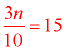2. Ten less than the quotient of a number and two is zero.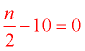3. Twelve more than the product of a number and two is thirty-six. 2n + 12 = 36 4. Represent the cost of access to a movie web site which charges a \$5 fee for the first month, and then \$28 a month for continued access after the first month. Numerical Example: For 4 months the cost would be: Cost = \$5 + \$28•(4-1). Replace 4 with m for number of months. c = cost, m = months c = 5 + 28(m - 1) 5. If chocolate doughnuts cost \$1.10 and chocolate chip muffins cost \$2.25, how many of each can you purchase for \$20? Numerical Example: The cost of 3 doughnuts and 2 muffins will be: Cost = \$1.50•3 + \$2.25• 2. Replace 3 with d for doughnuts, 2 with m for muffins, and Cost with \$20. d =doughnuts, m = muffins 20 = 1.50d + 2.25m 6. Express the area of a rectangle whose length is twice its width decreased by 6. Numerical Example: If the width is 10, the length is 14, then the area, A = 10 • 14. Just replace 10 with w for width, and 14 with 2w - 6 for the length. A = area, w = width A = w • (2w - 6)